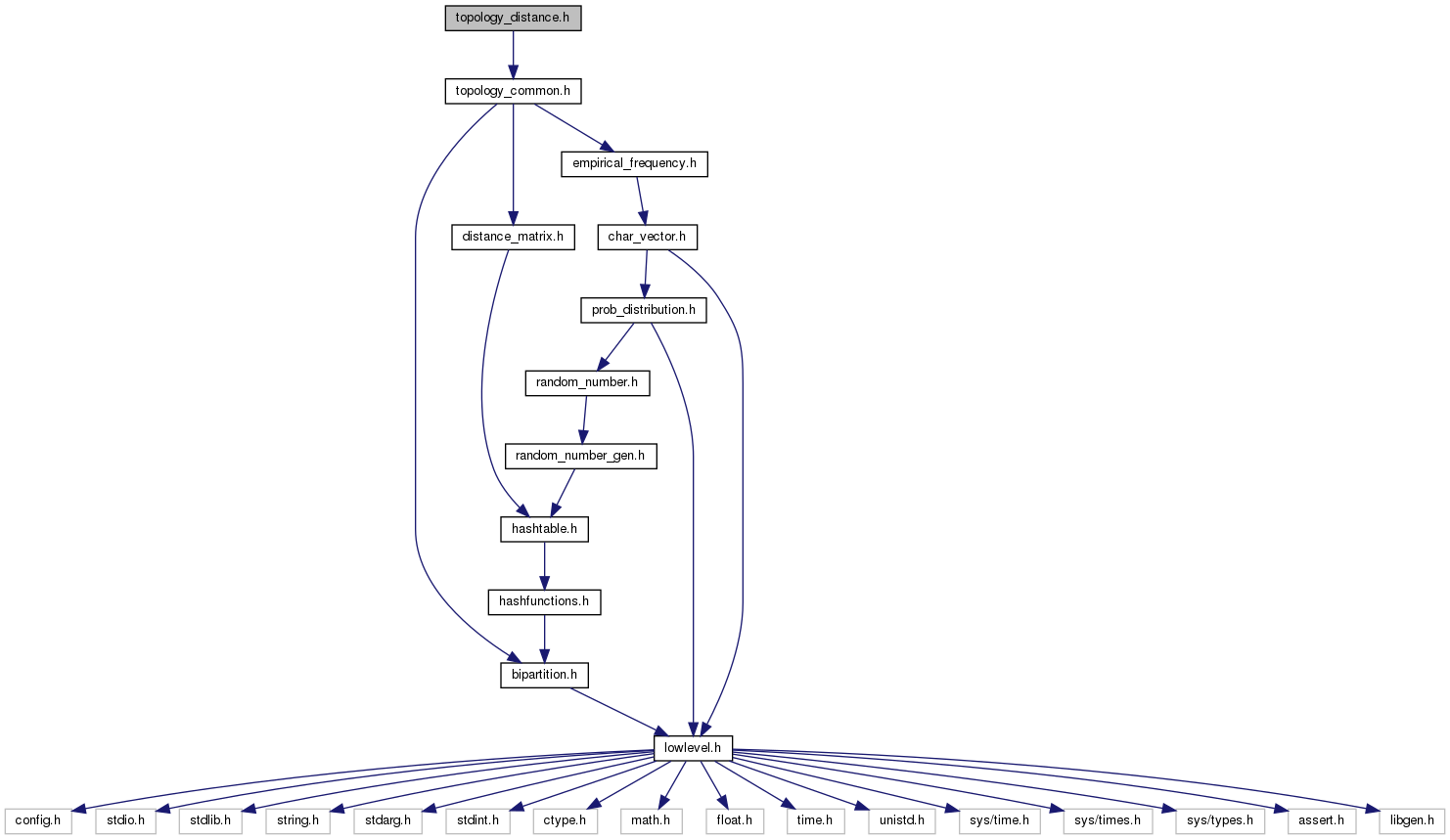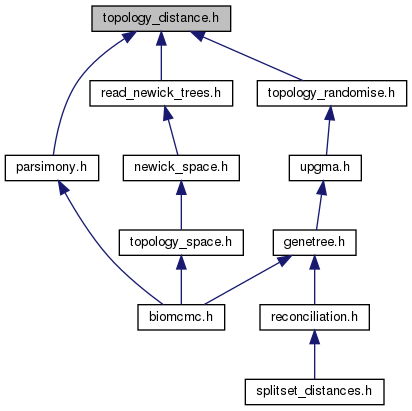biomcmc-lib  0.1 low level library for phylogenetic analysis
topology_distance.h File Reference

branch length operations on topologies, including patristic distances More...

`#include "topology_common.h"`
Include dependency graph for topology_distance.h:This graph shows which files directly or indirectly include this file:Go to the source code of this file.

## Functions

distance_matrix new_distance_matrix_for_topology (int nleaves)
allocate memory for a new distance_matrix that will be used on topologies

void fill_distance_matrix_from_topology (distance_matrix dist, topology tree, double *blen, bool use_upper)
fill in distance_matrix with the patristic distances from topology (can be used with distinct branch length vectors to fill upper and lower diagonals

void patristic_distances_from_topology_to_vectors (topology tree, double **dist, double *scaling, int n_dists, double tolerance)
calculates rescaled patristic distances returning up to 6 distinct 1D vectors #dist (externally allocated) The 'tolerance' is the minimum branch length to be considered a multifurcation (length zero)

int * create_vector_with_idx_leaves_below_for_patristic (topology tree)
similar to an Euler tour, has list of leaves below each node

void estimate_topology_branch_lengths_from_distances (topology tree, double *dist)

double * new_topology_branch_lengths_from_distances (topology tree, double *dist)

void correct_negative_branch_lengths_from_topology (topology t, double *blength)

## Detailed Description

branch length operations on topologies, including patristic distances